# 操作canvas：实时时钟制作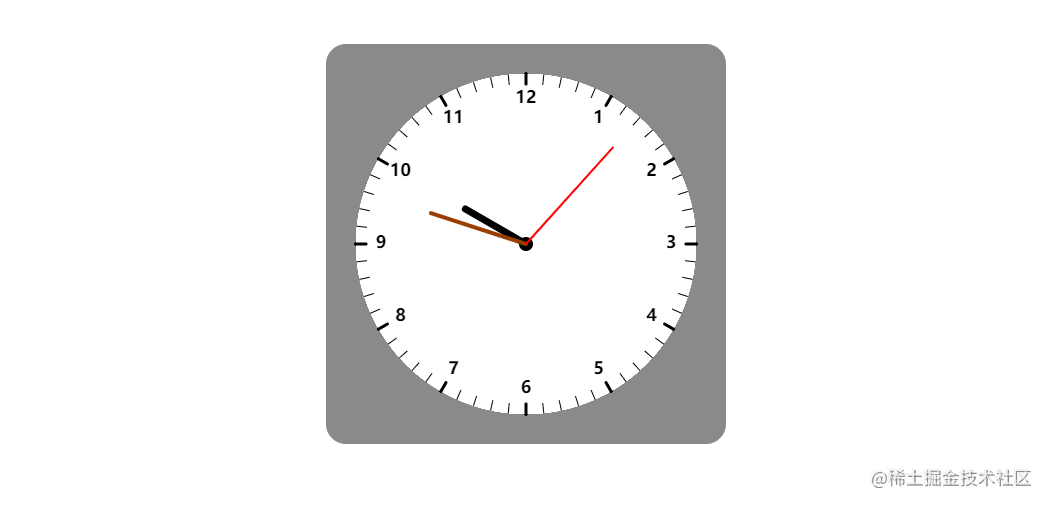#### 一、画制时钟

##### 1.绘制钟盘和钟盘圆心

``````    <style>
/* 时钟的样式 */
.clock {
display: block;
margin: 100px auto;
background: #ccc;
}
</style>

<!-- 画板 -->
<div class="clock">
<canvas width="400px" height="400px"></canvas>
</div>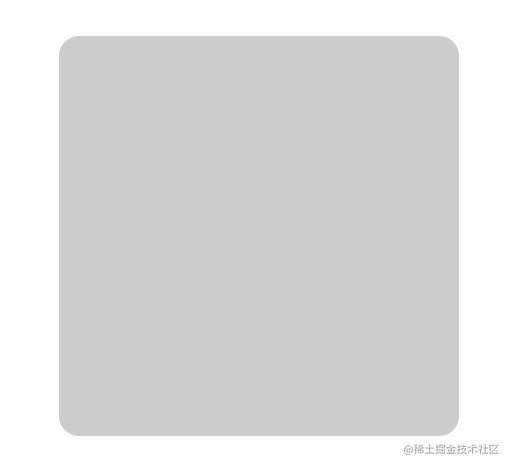html和css部分就弄好了，下面就要通过JavaScript操作canvas了，这里我们先要定义一下几个要使用到的变量：

`var canvas = document.querySelector("canvas"); var clock = canvas.getContext('2d');`

`clock.arc(x,y,r,sAngle,eAngle,counterclockwise)`函数画制时钟的圆环

x：数值（位于画板的x坐标）
y：数值（位于画板的y坐标）
r：数值（绘制圆弧的半径）
sAngle：弧度（绘制圆弧的起始弧度）
eAngle：弧度（绘制圆弧的结束弧度）
counterclockwise：布尔值（绘制圆弧是从顺时针还是逆时针）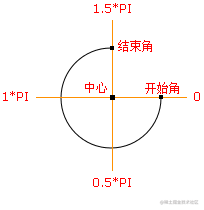``````// 画时钟的圆盘和钟盘的圆心
clock.beginPath();
clock.arc(200,200,clockWidth,0,2*Math.PI,false);
clock.fillStyle = "#fff";
clock.fill();
clock.beginPath();
clock.arc(200,200,7,0,2*Math.PI,false);
clock.fillStyle = '#000';
clock.fill();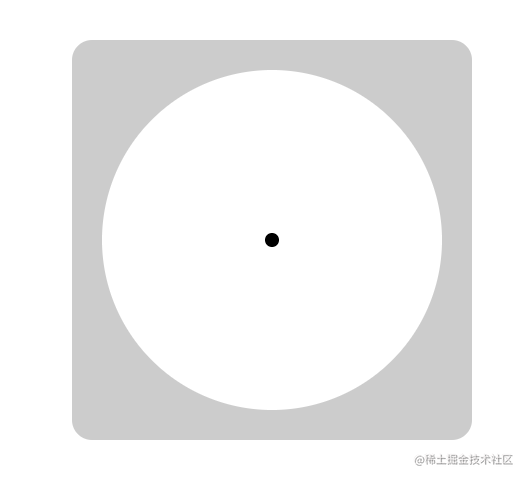##### 2.画制钟盘刻度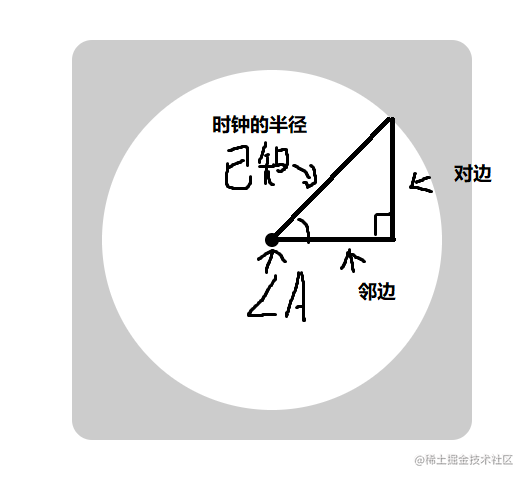``````// 画制线条函数
/*
start1: 起始横坐标
start2：起始纵坐标
end1：结束横坐标
end2：结束纵坐标
color：设置线条样式
*/
function dwLine(start1,start2,end1,end2,color="#000"){
clock.beginPath();
clock.moveTo(start1,start2);
clock.lineTo(end1,end2);
clock.strokeStyle = color;
clock.stroke();
clock.closePath();
}

// 画制时钟刻度
// 定义1°
var pi = Math.PI*2/360;

for(var i=1;i<=60;i++){
if(i%5==0){
clock.lineWidth = 3;
}
else {
clock.lineWidth = 1;
}
var x = Math.cos(pi*6*i);
var y = Math.sin(pi*6*i);
dwLine(200+r_clock*x,200+r_clock*y,200+(r_clock-10)*x,200+(r_clock-10)*y)
}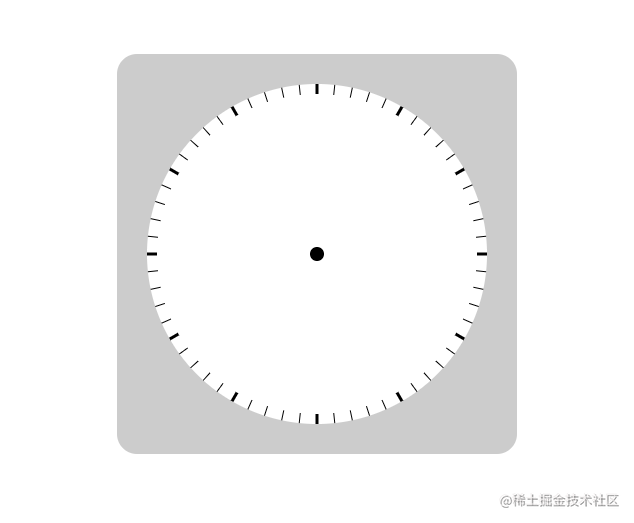##### 3.绘制时钟钟点数字

``````// 定义1°
var pi = Math.PI*2/360;

for(var i=1;i<=60;i++){
var x = Math.cos(pi*6*i-Math.PI/2);
var y = Math.sin(pi*6*i-Math.PI/2);
if(i%5==0){
clock.lineWidth = 3;
clock.textAlign = 'center';
clock.textBaseline = 'middle';
clock.font = 'bold 17px 微软雅黑';
clock.fillText(i/5,200+145*x,200+145*y);
}
else {
clock.lineWidth = 1;
}
dwLine(200+r_clock*x,200+r_clock*y,200+(r_clock-10)*x,200+(r_clock-10)*y)
}

1.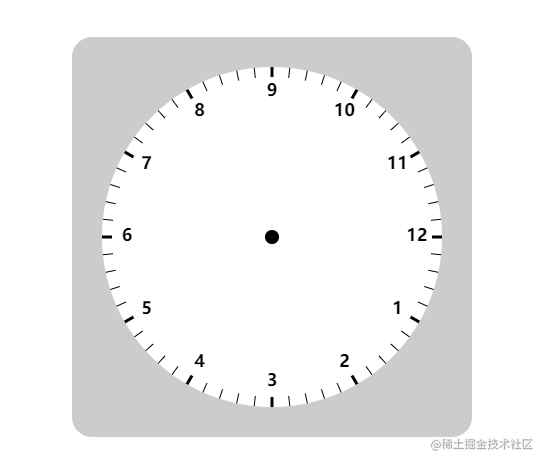2.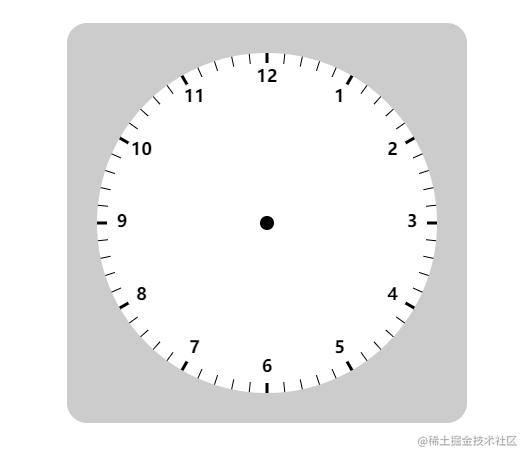#### 二.让时钟动起来

``````var date = new Date();
var h = date.getHours();
if(h>12){
h-=12;
}
var m = date.getMinutes();
var s = date.getSeconds();

``````// 定义1°
var pi = Math.PI*2/360;
clock.lineWidth = 7;
var x = Math.cos(pi*h*30-Math.PI/2);
var y = Math.sin(pi*h*30-Math.PI/2);
dwLine(200,200,200+r_hours*x,200+r_hours*y,'#000');

clock.lineWidth = 4;
var x = Math.cos(pi*m*6-Math.PI/2);
var y = Math.sin(pi*m*6-Math.PI/2);
dwLine(200,200,200+r_minute*x,200+r_minute*y,'rgb(151, 63, 0)');

clock.lineWidth = 2;
var x = Math.cos(pi*s*6-Math.PI/2);
var y = Math.sin(pi*s*6-Math.PI/2);
dwLine(200,200,200+r_second*x,200+r_second*y,'red');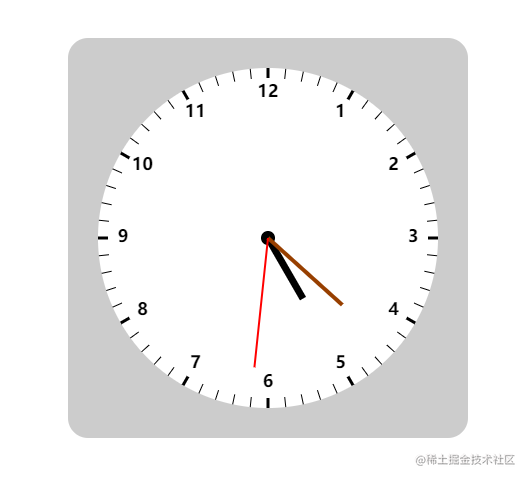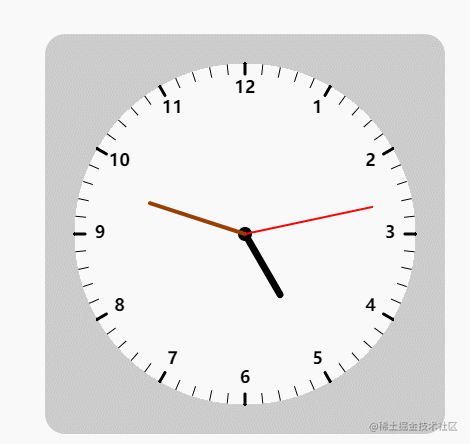HTML部分

``````<style>
/* 时钟的样式 */
canvas {
display: block;
margin: 100px auto;
background: #ccc;
}
</style>

<!-- 画板 -->
<div class="clock">
<canvas width="400px" height="400px"></canvas>
</div>

JS部分

``````// 基本变量
// 钟盘大小
var r_clock = 170;
// 时针长度：时分秒
var r_hours = 70;
var r_minute = 100;
var r_second = 130;
// 定义1°
var pi = Math.PI*2/360;
// 获取画板
var canvas = document.querySelector("canvas");
var clock = canvas.getContext('2d');

// 画线条函数
function dwLine(start1,start2,end1,end2,color="#000"){
clock.beginPath();
clock.moveTo(start1,start2);
clock.lineTo(end1,end2);
clock.strokeStyle = color;
clock.stroke();
clock.closePath();
clock.lineCap = 'round';
}

function time() {
// 绘制时钟的圆盘和钟盘的圆心
clock.beginPath();
clock.arc(200,200,r_clock,0,2*Math.PI,false);
clock.fillStyle = "#fff";
clock.fill();
clock.beginPath();
clock.arc(200,200,7,0,2*Math.PI,false);
clock.fillStyle = '#000';
clock.fill();

// 绘制时钟刻度
clock.strokeStyle = "#000";
for(var i=1;i<=60;i++){
var x = Math.cos(pi*6*i-Math.PI/2);
var y = Math.sin(pi*6*i-Math.PI/2);
if(i%5==0){
clock.lineWidth = 3;
// 绘制时钟数字
clock.textAlign = 'center';
clock.textBaseline = 'middle';
clock.font = 'bold 17px 微软雅黑';
clock.fillText(i/5,200+145*x,200+145*y);
}
else {
clock.lineWidth = 1;
}
dwLine(200+r_clock*x,200+r_clock*y,200+(r_clock-10)*x,200+(r_clock-10)*y)
}

// 获取当前的时分秒
var date = new Date();
var h = date.getHours();
if(h>12){
h-=12;
}
var m = date.getMinutes();
var s = date.getSeconds();

// 绘制时针
clock.lineWidth = 7;
var x = Math.cos(pi*h*30-Math.PI/2);
var y = Math.sin(pi*h*30-Math.PI/2);
dwLine(200,200,200+r_hours*x,200+r_hours*y,'#000');

// 绘制分针
clock.lineWidth = 4;
var x = Math.cos(pi*m*6-Math.PI/2);
var y = Math.sin(pi*m*6-Math.PI/2);
dwLine(200,200,200+r_minute*x,200+r_minute*y,'rgb(151, 63, 0)');

// 绘制秒针
clock.lineWidth = 2;
var x = Math.cos(pi*s*6-Math.PI/2);
var y = Math.sin(pi*s*6-Math.PI/2);
dwLine(200,200,200+r_second*x,200+r_second*y,'red');
}

time();

setInterval(function(){
time();
},1000);